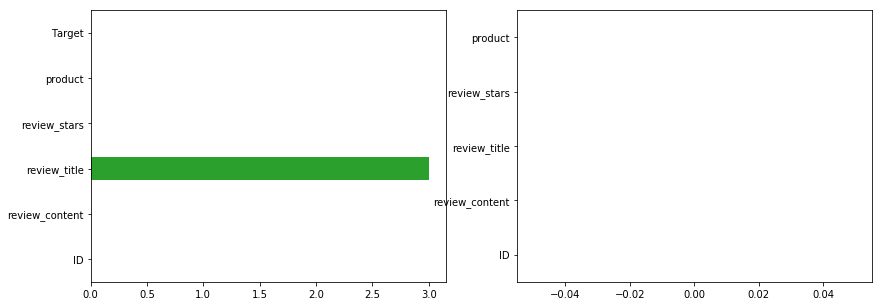In :
import os
import sys
import numpy as np
import pandas as pd
import matplotlib.pyplot as plt
import seaborn as sns
from wordcloud import WordCloud
from nltk.stem import WordNetLemmatizer
from sklearn.feature_extraction.text import CountVectorizer, TfidfVectorizer
%matplotlib inline
# always reload modules marked with "%aimport"

# add the 'src' directory as one where we can import modules
src_dir = os.path.join(os.getcwd(), os.pardir, 'src')
sys.path.append(src_dir)

# import my method from the source code

In :
train, test = read_data(test=True)

In :
print(train.shape)

(60000, 6)

Out:
ID review_content review_title review_stars product Target
0 0 En appelant un acheteur pour demander si l'écr... La Police s'inscrit en acheteur privé sur Pric... 5 2fbb619e3606f9b7c213e858a109cda771aa2c47ce50d5... 0
1 1 Alors, là, on a affaire au plus grand Navet ja... Chef D'Oeuvre Absolu en vue... 5 7b56d9d378d9e999d293f301ac43d044cd7b4786d09afb... 1
2 2 Effet garanti sur la terrase. Ils donnent immé... Effet garanti sur la terrase. Ils donnent immé... 3 7b37bf5dcb2fafd9229897910318a7dfa11a04ca36893c... 0
3 3 tres bon rapport qualite prix tre pratique en ... bon produit 4 77d2dbd504b933ab3aaf7cb0cd81c22f7c3549012f4f88... 1
4 4 Ordinateur de bureau trés bien pour quelqu'un ... Apple Power MAC G4 3 f574512e7d2dd1dd73c7f8f804bf16f14c932c5651a01b... 1

## Number of target classes¶

In :
fig, axr = plt.subplots(1,2, figsize=(14,5))
sns.countplot(train['Target'], ax=axr)
train.groupby(['Target', 'review_stars']).size().unstack('Target').plot(kind='bar', stacked=True, ax=axr)

Out:
<matplotlib.axes._subplots.AxesSubplot at 0x7f44f489ea20>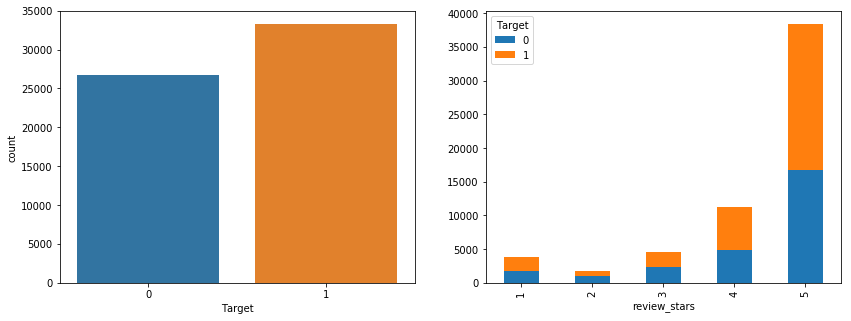• Not imbalanced classes
• All classes of review starts have positive and negative samples

## Duplicates¶

In :
sns.countplot(y=train['product'], order=train['product'].value_counts()[:20].index)

Out:
<matplotlib.axes._subplots.AxesSubplot at 0x7f8f260fe550>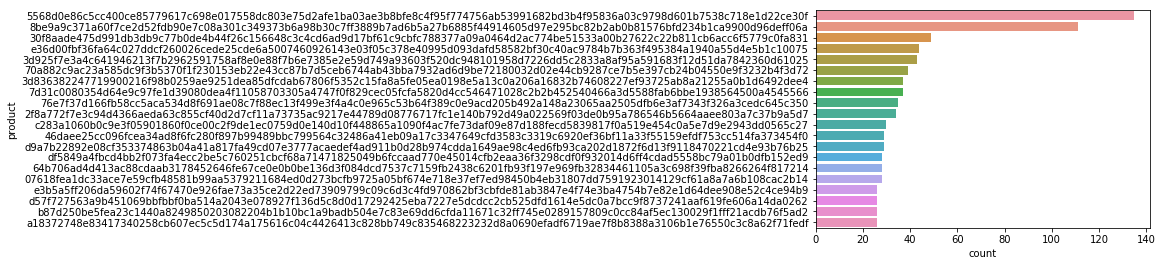In :
"Appear only one {}, More than one time {}".format((train.groupby('product').size() == 1).sum(),
(train.duplicated('product', keep=False).sum()))

Out:
'Appear only one 40068, More than one time 19932'
In :
train[train['Target'] == 0].groupby('product').size().plot(kind='hist', logy=True, bins=100, figsize=(10,5), alpha=0.5,
label='0. Mean product reviews : {:.2f}'.format(train[train['Target'] == 0].groupby('product').size().mean()))
train[train['Target'] == 1].groupby('product').size().plot(kind='hist', logy=True, bins=100, figsize=(10,5), alpha=0.5,
label='1. Mean product reviews : {:.2f}'.format(train[train['Target'] == 1].groupby('product').size().mean()))
plt.legend(title='Target')
plt.xlabel('Product reviews per product')
plt.ylabel('Number of products')

Out:
Text(0,0.5,'Number of products')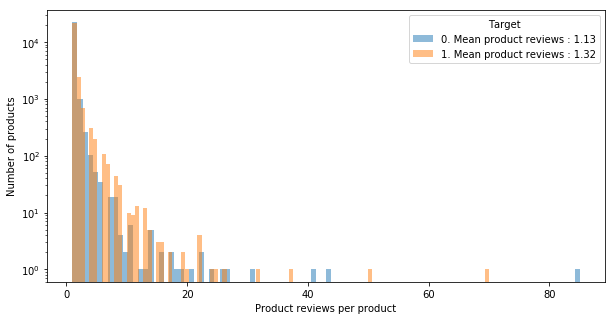# Size of posts¶

In :
fig, axr = plt.subplots(1,2, figsize=(14,5))
sns.distplot(np.log(train[train['Target'] == 0]['review_content'].apply(lambda x: len(x.split()))), label='Negative', ax=axr)
sns.distplot(np.log(train[train['Target'] == 1]['review_content'].apply(lambda x: len(x.split()))), label='Positive', ax=axr)
axr.legend()

sns.distplot(np.log(train[train['Target'] == 0]['review_title'].dropna().apply(lambda x: len(x.split() if x else np.NaN))), label='Negative', ax=axr)
sns.distplot(np.log(train[train['Target'] == 1]['review_title'].dropna().apply(lambda x: len(x.split() if x else np.NaN))), label='Positive', ax=axr)
axr.legend()

Out:
<matplotlib.legend.Legend at 0x7f8f090c07b8>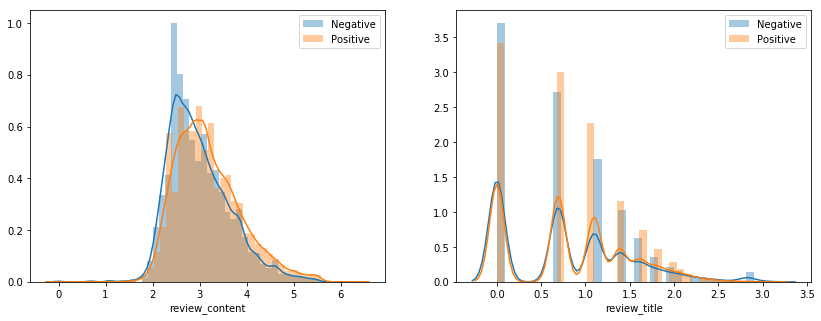• Large review post tend to be more positive

# Words title¶

In :
plt.figure(figsize=(14,4))
plt.title('Uncleaned words from rewiews')
sns.countplot(train['review_title'].str.split(expand=True).unstack(),
order=train['review_title'].str.split(expand=True).unstack().value_counts()[:20].index)

Out:
<matplotlib.axes._subplots.AxesSubplot at 0x7f8f26e73240>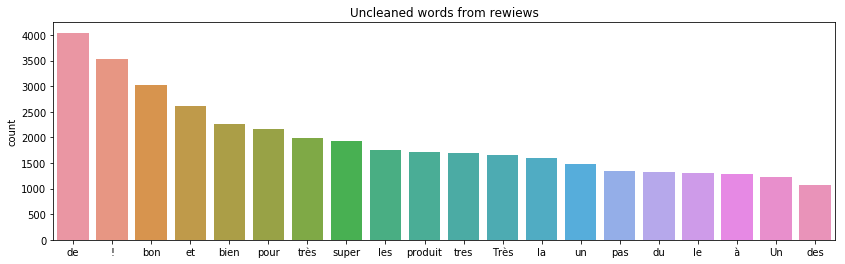In :
with open('../data/external/fr-stopwords.txt') as fp:

In :
positive = train[train['Target'] == 1]['review_title'].dropna().values
negative = train[train['Target'] == 0]['review_title'].dropna().values

In :
# The wordcloud of Cthulhu/squidy thing for HP Lovecraft
fig, axr = plt.subplots(1,2, figsize=(16,13))
wcP = WordCloud(background_color="white", max_words=10000,
stopwords=stopwords, max_font_size= 40)
wcN = WordCloud(background_color="black", max_words=10000,
stopwords=stopwords, max_font_size= 40)
wcP.generate(" ".join(positive))
wcN.generate(" ".join(negative))
#plt.title("HP Lovecraft (Cthulhu-Squidy)", fontsize=20)
axr.set_title('Positive')
axr.set_title('Negative')
axr.imshow(wcP.recolor( colormap= 'viridis' , random_state=17), alpha=0.98)
axr.imshow(wcN.recolor( colormap= 'Pastel1_r' , random_state=17), alpha=0.98)
axr.axis('off'); axr.axis('off')

Out:
(-0.5, 399.5, 199.5, -0.5)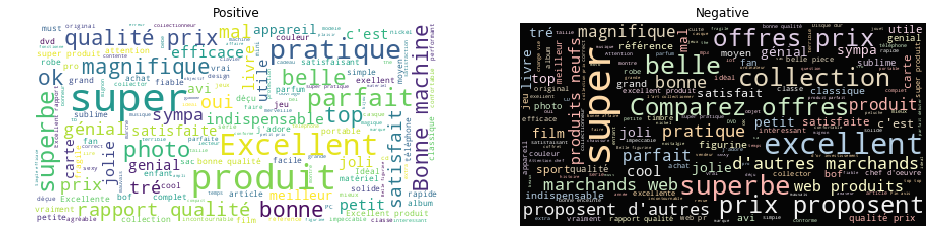# Words content¶

In :
plt.figure(figsize=(14,4))
plt.title('Uncleaned words from rewiews')
sns.countplot(train['review_content'].str.split(expand=True).unstack(),
order=train['review_content'].str.split(expand=True).unstack().value_counts()[:20].index)

ERROR:root:Internal Python error in the inspect module.
Below is the traceback from this internal error.


Traceback (most recent call last):
File "/home/cris/.virtualenvs/cdiscount/lib/python3.6/site-packages/IPython/core/interactiveshell.py", line 2910, in run_code
exec(code_obj, self.user_global_ns, self.user_ns)
File "<ipython-input-33-4d58ad6b10a4>", line 4, in <module>
order=train['review_content'].str.split(expand=True).unstack().value_counts()[:20].index)
File "/home/cris/.virtualenvs/cdiscount/lib/python3.6/site-packages/seaborn/categorical.py", line 3359, in countplot
errcolor, errwidth, capsize, dodge)
File "/home/cris/.virtualenvs/cdiscount/lib/python3.6/site-packages/seaborn/categorical.py", line 1596, in __init__
order, hue_order, units)
File "/home/cris/.virtualenvs/cdiscount/lib/python3.6/site-packages/seaborn/categorical.py", line 203, in establish_variables
group_names)
File "/home/cris/.virtualenvs/cdiscount/lib/python3.6/site-packages/seaborn/categorical.py", line 249, in _group_longform
g_vals = np.asarray(grouped_vals.get_group(g))
File "/home/cris/.virtualenvs/cdiscount/lib/python3.6/site-packages/pandas/core/groupby.py", line 763, in get_group
inds = self._get_index(name)
File "/home/cris/.virtualenvs/cdiscount/lib/python3.6/site-packages/pandas/core/groupby.py", line 609, in _get_index
return self._get_indices([name])
File "/home/cris/.virtualenvs/cdiscount/lib/python3.6/site-packages/pandas/core/groupby.py", line 576, in _get_indices
if len(self.indices) > 0:
File "/home/cris/.virtualenvs/cdiscount/lib/python3.6/site-packages/pandas/core/groupby.py", line 555, in indices
return self.grouper.indices
File "pandas/_libs/properties.pyx", line 38, in pandas._libs.properties.cache_readonly.__get__
File "/home/cris/.virtualenvs/cdiscount/lib/python3.6/site-packages/pandas/core/groupby.py", line 1980, in indices
return self.groupings.indices
File "pandas/_libs/properties.pyx", line 38, in pandas._libs.properties.cache_readonly.__get__
File "/home/cris/.virtualenvs/cdiscount/lib/python3.6/site-packages/pandas/core/groupby.py", line 2744, in indices
values = _ensure_categorical(self.grouper)
File "/home/cris/.virtualenvs/cdiscount/lib/python3.6/site-packages/pandas/core/dtypes/common.py", line 81, in _ensure_categorical
arr = Categorical(arr)
File "/home/cris/.virtualenvs/cdiscount/lib/python3.6/site-packages/pandas/core/categorical.py", line 330, in __init__
codes, categories = factorize(values, sort=True)
File "/home/cris/.virtualenvs/cdiscount/lib/python3.6/site-packages/pandas/core/algorithms.py", line 479, in factorize
assume_unique=True)
File "/home/cris/.virtualenvs/cdiscount/lib/python3.6/site-packages/pandas/core/sorting.py", line 477, in safe_sort
mask = (labels < -len(values)) | (labels >= len(values)) | \
KeyboardInterrupt

During handling of the above exception, another exception occurred:

Traceback (most recent call last):
File "/home/cris/.virtualenvs/cdiscount/lib/python3.6/site-packages/IPython/core/interactiveshell.py", line 1828, in showtraceback
stb = value._render_traceback_()
AttributeError: 'KeyboardInterrupt' object has no attribute '_render_traceback_'

During handling of the above exception, another exception occurred:

Traceback (most recent call last):
File "/home/cris/.virtualenvs/cdiscount/lib/python3.6/site-packages/IPython/core/ultratb.py", line 1090, in get_records
return _fixed_getinnerframes(etb, number_of_lines_of_context, tb_offset)
File "/home/cris/.virtualenvs/cdiscount/lib/python3.6/site-packages/IPython/core/ultratb.py", line 311, in wrapped
return f(*args, **kwargs)
File "/home/cris/.virtualenvs/cdiscount/lib/python3.6/site-packages/IPython/core/ultratb.py", line 345, in _fixed_getinnerframes
records = fix_frame_records_filenames(inspect.getinnerframes(etb, context))
File "/usr/lib64/python3.6/inspect.py", line 1483, in getinnerframes
frameinfo = (tb.tb_frame,) + getframeinfo(tb, context)
File "/usr/lib64/python3.6/inspect.py", line 1445, in getframeinfo
lines, lnum = findsource(frame)
File "/home/cris/.virtualenvs/cdiscount/lib/python3.6/site-packages/IPython/core/ultratb.py", line 177, in findsource
lines = linecache.getlines(file, globals_dict)
File "/home/cris/.virtualenvs/cdiscount/lib/python3.6/linecache.py", line 47, in getlines
return updatecache(filename, module_globals)
File "/home/cris/.virtualenvs/cdiscount/lib/python3.6/linecache.py", line 137, in updatecache
File "/home/cris/.virtualenvs/cdiscount/lib/python3.6/codecs.py", line 318, in decode
def decode(self, input, final=False):
KeyboardInterrupt

---------------------------------------------------------------------------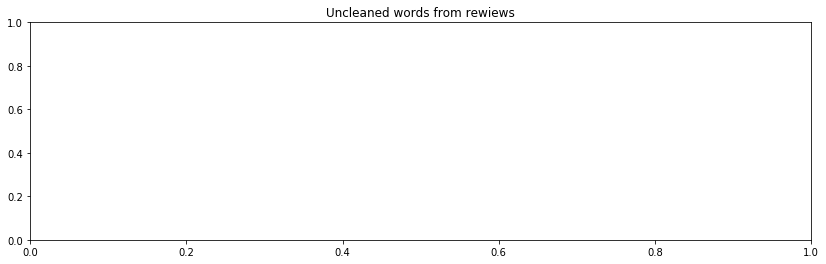In :
positive = train[train['Target'] == 1]['review_content'].dropna().values
negative = train[train['Target'] == 0]['review_content'].dropna().values

# The wordcloud of Cthulhu/squidy thing for HP Lovecraft
fig, axr = plt.subplots(1,2, figsize=(16,13))
wcP = WordCloud(background_color="white", max_words=10000,
stopwords=stopwords, max_font_size= 40)
wcN = WordCloud(background_color="black", max_words=10000,
stopwords=stopwords, max_font_size= 40)
wcP.generate(" ".join(positive))
wcN.generate(" ".join(negative))
#plt.title("HP Lovecraft (Cthulhu-Squidy)", fontsize=20)
axr.set_title('Positive')
axr.set_title('Negative')
axr.imshow(wcP.recolor( colormap= 'viridis' , random_state=17), alpha=0.98)
axr.imshow(wcN.recolor( colormap= 'Pastel1_r' , random_state=17), alpha=0.98)
axr.axis('off'); axr.axis('off')

Out:
(-0.5, 399.5, 199.5, -0.5)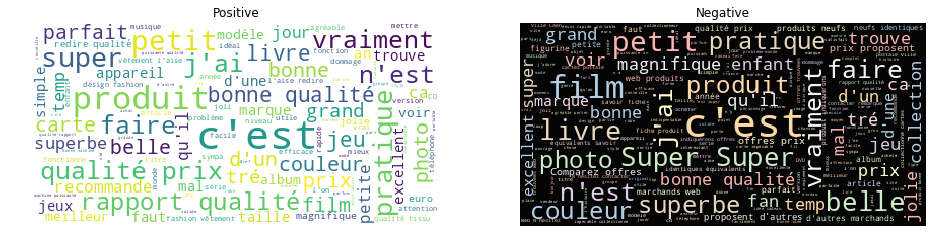## Lemma¶

In :
import nltk

[nltk_data] Downloading package wordnet to /home/cris/nltk_data...
[nltk_data]   Package wordnet is already up-to-date!

Out:
True
In :
lemm = WordNetLemmatizer()
class LemmaCountVectorizer(CountVectorizer):
def build_analyzer(self):
analyzer = super(LemmaCountVectorizer, self).build_analyzer()
return lambda doc: (lemm.lemmatize(w) for w in analyzer(doc))

In :
def get_lemmas(text):
tf_vectorizer = LemmaCountVectorizer(max_df=0.95,
min_df=2,
stop_words=stopwords,
decode_error='ignore')
tf = tf_vectorizer.fit_transform(text)
feature_names = tf_vectorizer.get_feature_names()
count_vec = np.asarray(tf.sum(axis=0)).ravel()
zipped = list(zip(feature_names, count_vec))
x,y = (list(x) for x in zip(*sorted(zipped, key=lambda x: x, reverse=True)))
return x,y


In :
# Storing the entire training text in a list
text_pos = list(train[train['Target'] == 1]['review_content'].dropna().values)
text_neg = list(train[train['Target'] == 0]['review_content'].dropna().values)

In :
x_pos, y_pos = get_lemmas(text_pos)
x_neg, y_neg = get_lemmas(text_neg)

In :
fig, axr = plt.subplots(2,figsize=(15,5))
axr.set_title('Cleaned words from rewiews')
sns.barplot(x=x_pos[0:20], y=y_pos[0:20], ax=axr, label='Positive')
axr.legend()
sns.barplot(x=x_neg[0:20], y=y_neg[0:20], ax=axr, label='Negative')
axr.legend()

Out:
<matplotlib.legend.Legend at 0x7f44db9975c0>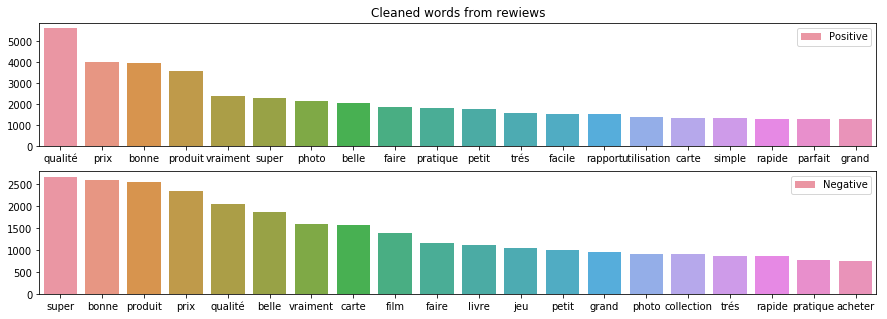# Tfidf¶

In :
def get_tfidf(text):
vectorizer = TfidfVectorizer(min_df=10, max_features=10000, ngram_range=(1, 2))
vz = vectorizer.fit_transform(list(text))
tfidf = dict(zip(vectorizer.get_feature_names(), vectorizer.idf_))
tfidf = pd.DataFrame(columns=['tfidf']).from_dict(dict(tfidf), orient='index')
tfidf.columns = ['tfidf']
return tfidf

In :
vectorizer = TfidfVectorizer(min_df=10, max_features=10000, ngram_range=(1, 2))
vz = vectorizer.fit_transform(list(text))

In :
tfidf = dict(zip(vectorizer.get_feature_names(), vectorizer.idf_))
tfidf = pd.DataFrame(columns=['tfidf']).from_dict(dict(tfidf), orient='index')
tfidf.columns = ['tfidf']

In :
tfidf_pos = get_tfidf(text_pos)
tfidf_neg = get_tfidf(text_neg)

In :
fig, axr = plt.subplots(2,figsize=(19,5))
axr.set_title('Tfidf from review content')
sns.barplot(x=df.index, y=df.tfidf, ax=axr, label='Positive')
axr.set_ylim(8,9.5)
axr.legend()
sns.barplot(x=df.index, y=df.tfidf, ax=axr, label='Negative')
axr.set_ylim(8,9.5)
axr.legend()

Out:
<matplotlib.legend.Legend at 0x7f44e4909630>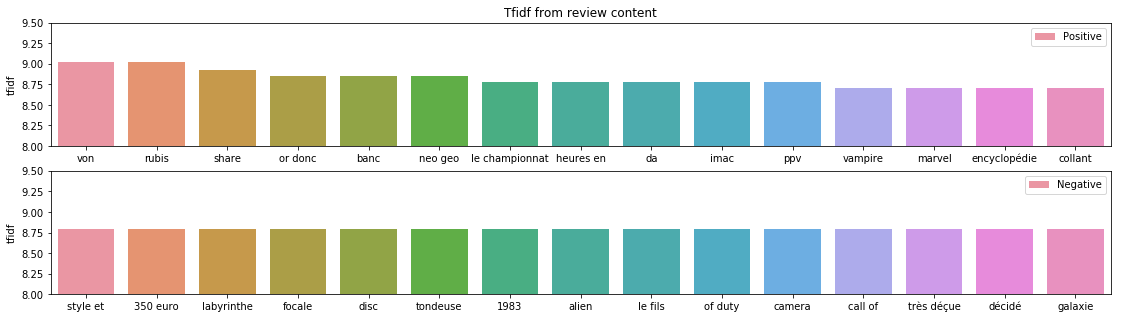In :
train['review_title'].dropna().str.split().apply(lambda x: [item for item in x if item not in stopwords])


# Missing values¶

In :
fig, axr = plt.subplots(1,2,figsize=(14,5))
train.apply(lambda col: col.isnull().sum(), axis=0).plot(kind='barh', ax=axr)
test.apply(lambda col: col.isnull().sum(), axis=0).plot(kind='barh', ax=axr)

Out:
<matplotlib.axes._subplots.AxesSubplot at 0x7f8f26bc9240>